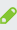# 根据七个大学排行榜统计得出最新大学排名

根据国内几个大学排行榜统计得出新的排名顺序：

(1)武书连2012；(2)校友会2012；(3)人大2012；(4)高等教育观察2012;(5)武汉大学2012,(6)网大2011，(7)上交大2011

1北京大学=2+1+1+1+1+2+2=10

2清华大学=3+2+2+2+2+1+1=13

3浙江大学=1+4+5+4+3+3+4=24

4复旦大学=5+3+3+3+4+6.5+6=30.5

5上海交大=4+5+7+7+5+6.5+5=39.5

6南京大学=6+6+6+6+6+5+7=42

7中国科技大学=17+9+8+5+17+4+3=63

8武汉大学=7+8+14+10+7+14.5+12=72.5

9华科=11+11+17+12+8+14.5+10=83.5

10南开=14+15+10+9+18+10.5+8=84.5

11中山大学=8+7+16+11+9+18+16=69=85

12哈工大=10+18+12+15+14+8.5+9=86.5

13人大=18+13+4+16+15+8.5+17=91.5

14北师大=22+17+9+13+16+12+14=103

15西安交大=15+19+13+8+13+14.5+24=106.5

16四川大学=9+12+21+23+10+14.5+25=114.5

17吉林大学=12+10+30+26+11+20+13=122

18北航大学=27+24+11+14+25+10.5+15=126.5

19天津大学=20+23+15+17+22+19+18=134

20厦门大学=23+20+18+19+21+17+20=138

21山东大学=12+14+25+27+12+26+27=143

22东南大学=19+22+22+18+20+23.5+21=145.5

23中南大学=16+16+23+24+19+23.5+28=149.5

24同济大学=25+21+19+25+23+28.5+22=163.5

25农业大学=31+27+20+21+29+27+11=166

26大连理工=21+25+26+22+26+23.5+23=166.5

注：本数据来源于ｅ度论坛，仅供参考！

•提建议：jszsorg#126.com（请把#换成@）